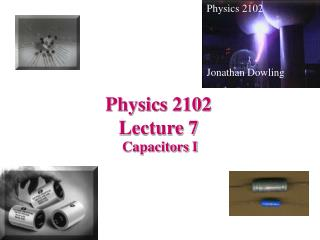DownloadDownload PresentationPhysics 2102 Lecture 7

# Physics 2102 Lecture 7

Download Presentation## Physics 2102 Lecture 7

- - - - - - - - - - - - - - - - - - - - - - - - - - - E N D - - - - - - - - - - - - - - - - - - - - - - - - - - -
##### Presentation Transcript

1. Physics 2102 Jonathan Dowling Physics 2102 Lecture 7 Capacitors I

2. Capacitors and Capacitance Capacitor: any two conductors, one with charge +Q, other with charge –Q –Q Potential DIFFERENCE between conductors = V +Q Uses: storing and releasing electric charge/energy. Most electronic capacitors: micro-Farads (mF), pico-Farads (pF) — 10–12 F New technology: compact 1 F capacitors Q = CV where C = capacitance Units of capacitance: Farad (F) = Coulomb/Volt

3. +Q –Q Capacitance • Capacitance depends only on GEOMETRICAL factors and on the MATERIAL that separates the two conductors • e.g. Area of conductors, separation, whether the space in between is filled with air, plastic, etc. (We first focus on capacitors where gap is filled by AIR!)

4. Electrolytic (1940-70) Electrolytic (new) Paper (1940-70) Capacitors Variable air, mica Mica (1930-50) Ceramic (1930 on) Tantalum (1980 on)

5. Parallel Plate Capacitor We want capacitance: C=Q/V Area of each plate = A Separation = d charge/area = s = Q/A E field between the plates: (Gauss’ Law) Relate E to potential difference V: +Q -Q What is the capacitance C ?

7. Parallel Plate Capacitor — Example • A huge parallel plate capacitor consists of two square metal plates of side 50 cm, separated by an air gap of 1 mm • What is the capacitance? C = e0A/d = (8.85 x 10–12 F/m)(0.25 m2)/(0.001 m) = 2.21 x 10–9 F (Very Small!!) Lesson: difficult to get large values of capacitance without special tricks!

8. –Q +Q Isolated Parallel Plate Capacitor • A parallel plate capacitor of capacitance C is charged using a battery. • Charge = Q, potential difference = V. • Battery is then disconnected. • If the plate separation is INCREASED, does Potential DifferenceV: (a) Increase? (b) Remain the same? (c) Decrease? • Q is fixed! • C decreases (=e0A/d) • Q=CV; V increases.

9. –Q +Q Parallel Plate Capacitor & Battery • A parallel plate capacitor of capacitance C is charged using a battery. • Charge = Q, potential difference = V. • Plate separation is INCREASED while battery remains connected. Does the Electric Field Inside: (a) Increase? (b) Remain the Same? (c) Decrease? • V is fixed by battery! • C decreases (=e0A/d) • Q=CV; Q decreases • E = Q/ e0A decreases

10. Spherical Capacitor What is the electric field inside the capacitor? (Gauss’ Law) Radius of outer plate = b Radius of inner plate = a Concentric spherical shells: Charge +Q on inner shell, –Q on outer shell Relate E to potential difference between the plates:

11. Spherical Capacitor What is the capacitance? C = Q/V = Radius of outer plate = b Radius of inner plate = a Concentric spherical shells: Charge +Q on inner shell, –Q on outer shell Isolated sphere: let b >> a,

12. Cylindrical Capacitor What is the electric field in between the plates? Gauss’ Law! Radius of outer plate = b Radius of inner plate = a Length of capacitor = L +Q on inner rod, –Q on outer shell Relate E to potential difference between the plates: cylindrical Gaussian surface of radius r

13. Summary • Any two charged conductors form a capacitor. • Capacitance : C= Q/V • Simple Capacitors:Parallel plates: C = e0 A/dSpherical: C = 4pe0 ab/(b-a)Cylindrical: C = 2pe0 L/ln(b/a)]

14. C1 Q1 A B C2 Q2 D C Ceq Qtotal Capacitors in Parallel • A wire is an equipotential surface! • Capacitors in parallel have SAME potential difference but NOT ALWAYS same charge! • VAB = VCD = V • Qtotal = Q1 + Q2 • CeqV = C1V + C2V • Ceq = C1 + C2 • Equivalent parallel capacitance = sum of capacitances

15. Q1 Q2 B C A C1 C2 Q Ceq Capacitors in Series • Q1 = Q2 = Q (WHY??) • VAC = VAB + VBC • SERIES: • Q is same for all capacitors • Total potential difference = sum of V